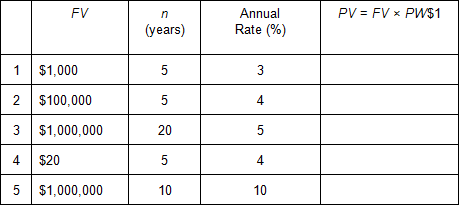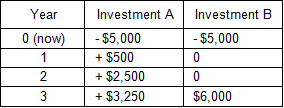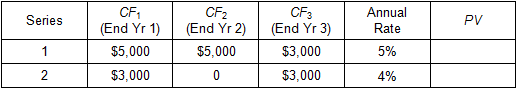Time Value of Money - Six Functions of a Dollar
Using Assessors Handbook Section 505 (Capitalization Formulas and Tables)
Appraisal Training: Self-Paced Online Learning Session

# Lesson 3: Present Worth of \$1

In order to solve the following problems you will need to refer to AH 505 to look up the factors in the compound interest tables. Before you start, please print the problems using the "Print Questions" button below so you can work through the problems on your own. After you work through the problem, the solution can be viewed by clicking on the blue plus sign immediately following the question. Additionally, when you have completed all the problems, you may print all of the solutions using the "Print Questions with Answers" button below.

## Problem 1

Calculate the present value (PV) of each future value (FV) listed below using the present worth of \$1 (PW\$1) factors in AH 505:## Problem 2

Assume that you would like to have \$500,000 saved by the time you reach 50 and that you are 30 years old today. If you can earn an annual interest rate of 8%, how much should you invest today to reach your goal?

## Problem 3

You would like to be able to withdraw \$10,000 at the end of 5 years, and another \$10,000 at the end of 10 years. If you can earn an annual interest rate of 5% on a deposit, how much should you deposit today?

## Problem 4

Mr. Smith wants to borrow money from you to finance his new business. He promises to repay you in two installments: a payment of \$5,000 two years from today, and a second payment of \$10,000 four years from today. If the annual interest rate is 10%, how much would you lend Mr. Smith today?

## Problem 5

Two investments A and B have the following annual cash flows (CF). Which investment is preferred, given an annual interest rate of 5%?
(The initial cash flows are negative, indicating outflows, and the subsequent cash flows are positive, indicating inflows. All inflows occur at the end of the year.)## Problem 6

Assuming all cash flows (CF) occur at the end of each year, determine the present values of each of the following two series of cash flows: